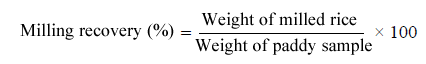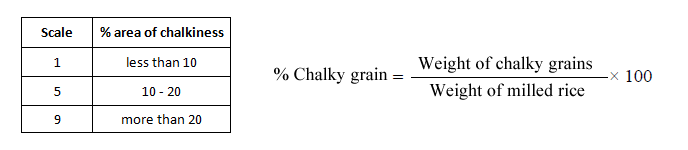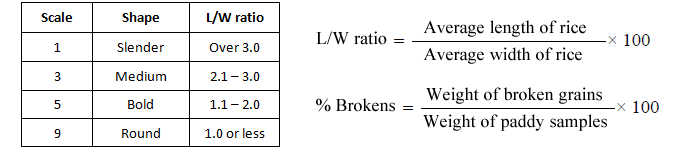Measuring white rice quality

What are the properties of milled rice that should be measured?

 1. Moisture2. Grain dimensions3. 1000-grain weight4. Milling degree5. Milling recovery 6. Dockage7. Head rice and broken grains8. Chalkiness9. Whiteness10. Grain shape

Measurements

Milling degree

Milling degree is computed on the basis of the amount of bran removed from the brown rice. To obtain the weight of brown rice, dehull the paddy samples using laboratory huller. Estimate the milling degree using the following equation:Milling recovery

Using an abrasive whitener, mill the dehulled samples. Compute milling recovery by dividing the weight of milled rice recovered by the weight of the paddy sample.Dockage

Select, segregate, and weigh the foreign matter. Record the number of unhulled grains collected from the sample. Determine the percentage of dockage of milled rice using the
equation:Using a grain grader, separate the broken grains from the whole grains. Compute the percentage of the milling recovery component using the following equation:Chalkiness

A visual rating of the chalky proportion of the grain is used to measure chalkiness based on the standard Evaluation System SES scale presented below: Select, segregate and weigh the chalky grains (SES Scale 9). Determine the % chalky grain using the equation:Whiteness

• Measure the grain whiteness using the Whiteness Meter.
• Separate and weigh yellow-fermented grains. Calculate the percentage of yellow/fermented grains using the formula:Grain Shape

Follow the procedure of determining grain shape of paddy. Based on the length to width ratio, the shape of the milled rice will be determined. The ISO Classification is as follows: